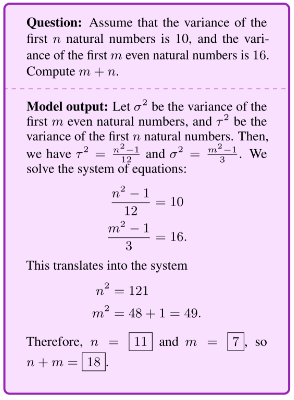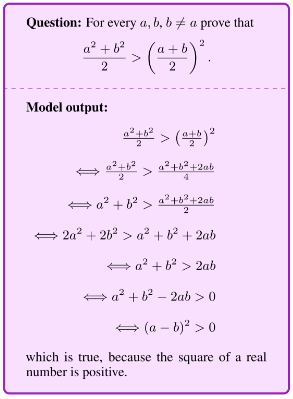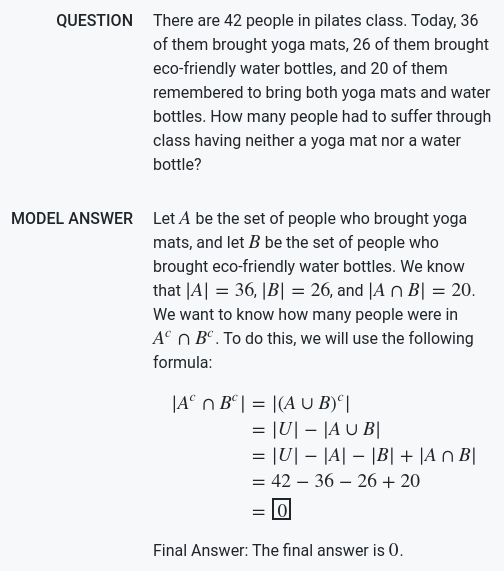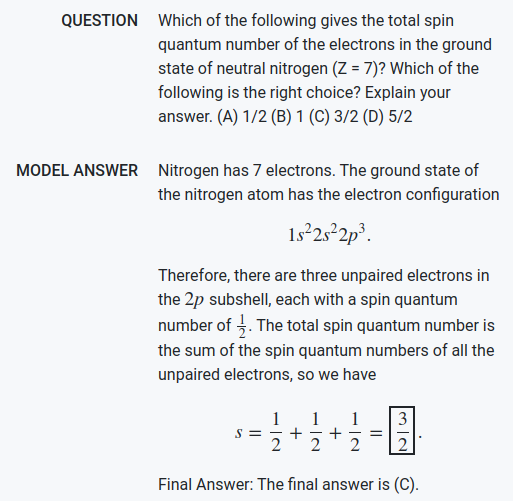# Google Minerva—Solving Quantitative Reasoning Problems with a Language Model

One of the classic challenges to artificial intelligence projects is solving “word problems” in mathematics and science. Word problems require extracting the logical and mathematical structure of the query from a written description, setting up the equations, the solving them and expressing the answer. This has been considered a test for “understanding” a query, rather than just regurgitating words from a large corpus of learned text, which I have called “bullshit generation”.

Google’s Minerva trained a language model similar to GPT-3 by building on an existing model called Pathways Language Model (PaLM) and then added an 118 Gb set of scientific papers from the arXiv preprint server and other sources. They then presented it with a series of questions from examinations taken by high school students around the world seeking higher education in science, engineering, and mathematics. Here is a problem from the Indian Joint Entrance Examination and Minerva’s verbatim answer.This is from the Polish National Math Exam.Here is a problem from the MATH Prealgebra Level 2 set which involves set theory.Let’s try one from the MMLU College Physics question set, this time multiple choice.Let’s throw one at it which requires trigonometry and calculus.

You can see many more, including ones where Minerva got the wrong answer, in the Minerva Sample Explorer on GitHub.

The research paper describing Minerva is “Solving Quantitative Reasoning Problems with Language Models”. Here is the abstract.

Language models have achieved remarkable performance on a wide range of tasks that require natural language understanding. Nevertheless, state-of-the-art models have generally struggled with tasks that require quantitative reasoning, such as solving mathematics, science, and engineering problems at the college level. To help close this gap, we introduce Minerva, a large language model pretrained on general natural language data and further trained on technical content. The model achieves state-of-the-art performance on technical benchmarks without the use of external tools. We also evaluate our model on over two hundred undergraduate-level problems in physics, biology, chemistry, economics, and other sciences that require quantitative reasoning, and find that the model can correctly answer nearly a third of them.

A popular description of Minerva, how it was built and trained, and its performance on various problem sets is posted on the Google AI Blog as “Minerva: Solving Quantitative Reasoning Problems with Language Models”.

Minerva is not, at the present, open to the public to submit queries.

6 Likes

This is super interesting and a nice counterpoint to the Wolfram Alpha approach.

Here is an example that works. And here is the pilates word problem from John’s post which does not pass through the word input filter.

5 Likes